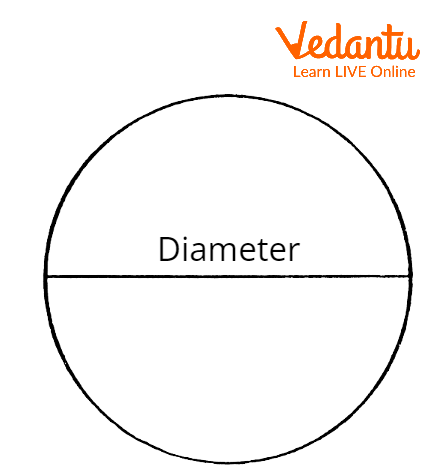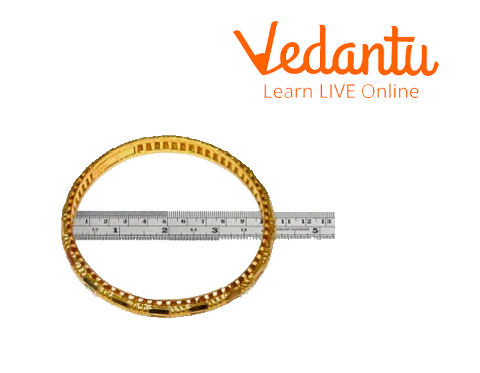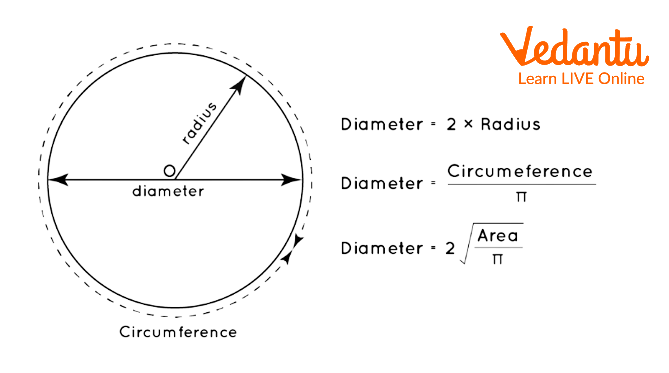Courses
Courses for Kids
Free study material
Free LIVE classes
More

# Diameter of a CircleLIVE
Join Vedantu’s FREE Mastercalss

## Introduction to Circle

We all have seen a circle and understand its shape and structure. Also, some of our daily used objects are circular in shape like plates, balls, mirrors, etc. However, did you know what is the diameter of a circle? You are aware of the term radius but have you ever heard about the diameter of a circle? Have you ever done a question associated with the diameter of a circle? The diameter of a circle is double the radius of the circle. It's the string line passing through the center of the circle connecting the two adjacent points on the circumference of the circle.

In this article, we are going to understand the concept of the diameter of a circle, what is diameter, the diameter symbol, and also the formula for the diameter of the circle.

## Diameter of a Circle

A circle is defined as the collection of points at a particular distance from the center point in a plane. The distance between the center and any point on the plane is termed radius. In the same manner, the distance from one point on the surface of the circle to the other point on its surface and passing through the center of the circle is termed its diameter.

In different words, the diameter is defined as double the radius of the circle.Diameter of a Circle

## What is Diameter?

In order to understand the concept of a diameter, take any circular object like a bangle or a plate. You'll see the circumference of a circle. Now, place a scale on the plate. This scale which joins the 2 adjacent points of the bangle or plate is termed the diameter of that bangle or plate.Diameter of a Bangle.

The concept of diameter is vital for numerous applications in arithmetic as well as in practical life also.

## Diameter Symbol

The symbol used for diameter in engineering is referred to as “phi” which is written as “⌀”. This symbol is employed to indicate the diameter of a circular section; as an example, "⌀20" means that the diameter of the circle is 20 units. The other symbol of diameter used is “d”.

## Formula for Diameter of a Circle

In order to calculate the diameter of a circle, we will use the formula :

d=2r

where, d is the diameter and r is the radius of the circle.

Now lets understand the concept behind this formula.

As you know that the radius of the circle is the distance between any of the points on the circumference and center. When we make 2 adjacent radii, they create a complete line and joins 2 parallel points on the circumference of the circle. This line is called the diameter.

Thus, double the value of radius is equal to the diameter of the circle.Formula for Diameter.

The different ways in which we can calculate the diameter of a circle are as follows :

If the radius of a circle is given , we will calculate the diameter of the circle as :

d=2r

where , d is the diameter of the circle and r is radius of circle.

When the circumference of the circle is given , the formula to calculate the diameter of circle is :

$d=\frac{C}{\pi }$

where , d is diameter , C is the circumference of circle and pi is the constant value.

When the area of the circle is given, the diameter of circle will be :

$d=\sqrt{\frac{4A}{\pi }}$

$d=2\sqrt{\frac{A}{\pi }}$

here , d is diameter of circle

A is the area of circle and pi is the contant value.

## Solved Problem

Q. Caculate the diameter of the circle when the circumference of circle is 78 cm .

Ans. Given : circumference of circle , C=78 cm.

To calculate : Diameter of circle , d .

We know that , when circumference of a circle is given , the formula used is :

$d=\frac{C}{\pi }$

$d=\frac{78}{3.14}$

$d=24.8\text{cm}$

Therefore , the diameter of circle is 24.8 cm.

## Summary

Two-dimensional shapes have only 2 dimensions, such as length and breadth, whereas 3D shapes need three dimensions. Along with length and breadth, 3D shapes have height. The shapes are defined using completely different terms length, height, area, etc. One such parameter that defines the shape circle is “Diameter”. We can conclude that the diameter is the longest chord of the circle.

Last updated date: 26th Sep 2023
Total views: 108k
Views today: 2.08k

## FAQs on Diameter of a Circle

1. What is the circumference of a circle?

The circumference of a circle is defined as the linear distance around it. In different words, if a circle is opened to form a line, then the length of that line will be the circle's circumference. it's the perimeter of a circle and therefore the formula used to calculate the circumference of a circle is C=2𝜋r. Here, C is the circle's circumference; r is the radius of the circle and 𝜋 is the constant value which may be 3.14.

2. Find the diameter of circle with radius 8 cm.

Diameter is double the value of radius and we know that if the radius is given, then the formula used to calculate the diameter of the circle is : d=2r.

Here , d is the diameter and r is the radius of circle.

Given value of radius , r = 8 cm.

According to the formula ,

$d=2r$

$d=2\times 8$

$d=16\text{cm}$

Therefore , Diameter of circle with radius of 8 cm is 16 cm.

3. Determine the diameter of circle whose area is 64cm2.

Given : area of circle , A = 64cm2.

If the circle’s area is given, then the formula used  to calculate the diameter of a circle is given by:

$d=\sqrt{\frac{4A}{\pi }}$

$d=2\sqrt{\frac{A}{\pi }}$

where A is the area of a circle and d is the diameter. By substituting the given values in the above formula, we get :

$d=2\sqrt{\frac{A}{\pi }}$

$d=2\sqrt{\frac{64}{3.14}}$

$d=2\times 4.51=9.02\text{cm}$

Therefore , the diameter of circle is 9.02 cm.